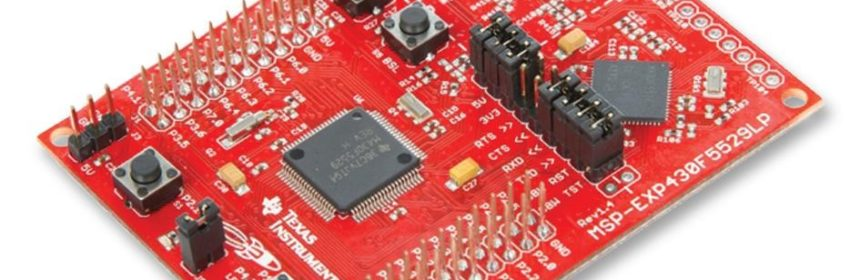## Tinkering TI MSP430F5529## Analogue Comparator – Comp_B+ Module

Although MSP430F5529 sports a good 12-bit ADC, it also packs a sophisticated analogue comparator called COMP_B+ that can be employed in a number of ways. As with any other embedded analogue comparator, it can be feed with external inputs as well as internal reference voltage generator – REF module. Additionally, this comparator has certain advanced features like software selectable RC filter, voltage hysteresis generator and timer input capture interlink. Positive and negative inputs can be shorted internally to remove any stray charge accumulation. Lastly, another attractive feature of COMP_B+ is its ultra-low power consumption.

Analogue comparators can be used in many cases. Some typical uses include

• voltage level monitor,
• measurement of capacitance,
• measurement of inductance,
• measurement of resistance,
• RC oscillators,
• noise remover,
• waveform converter,

### Code Example

```#include "driverlib.h"
#include "delay.h"

void clock_init(void);
void GPIO_init(void);
void Comp_B_Plus(void);

#pragma vector = COMP_B_VECTOR
__interrupt void Comp_B_ISR(void)
{
if(Comp_B_getInterruptStatus(COMP_B_BASE,
COMP_B_OUTPUT_FLAG))
{
GPIO_toggleOutputOnPin(GPIO_PORT_P4,
GPIO_PIN7);

Comp_B_clearInterrupt(COMP_B_BASE,
COMP_B_OUTPUT_FLAG);
}
}

void main(void)
{
WDT_A_hold(WDT_A_BASE);

clock_init();
GPIO_init();
Comp_B_Plus();

while(true)
{
};
}

void clock_init(void)
{
PMM_setVCore(PMM_CORE_LEVEL_3);

GPIO_setAsPeripheralModuleFunctionInputPin(GPIO_PORT_P5,
(GPIO_PIN4 | GPIO_PIN2));

GPIO_setAsPeripheralModuleFunctionOutputPin(GPIO_PORT_P5,
(GPIO_PIN5 | GPIO_PIN3));

UCS_setExternalClockSource(XT1_FREQ,
XT2_FREQ);

UCS_turnOnXT2(UCS_XT2_DRIVE_4MHZ_8MHZ);

UCS_turnOnLFXT1(UCS_XT1_DRIVE_0,
UCS_XCAP_3);

UCS_initClockSignal(UCS_MCLK,
UCS_XT2CLK_SELECT,
UCS_CLOCK_DIVIDER_1);

UCS_initClockSignal(UCS_SMCLK,
UCS_XT2CLK_SELECT,
UCS_CLOCK_DIVIDER_1);

UCS_initClockSignal(UCS_ACLK,
UCS_XT1CLK_SELECT,
UCS_CLOCK_DIVIDER_1);
}

void GPIO_init(void)
{
GPIO_setAsOutputPin(GPIO_PORT_P4,
GPIO_PIN7);

GPIO_setAsPeripheralModuleFunctionInputPin(GPIO_PORT_P6,
(GPIO_PIN0 + GPIO_PIN1));

GPIO_setAsPeripheralModuleFunctionOutputPin(GPIO_PORT_P1,
GPIO_PIN6);
}

void Comp_B_Plus(void)
{
Comp_B_initParam Comp_B_Param = {0};

Comp_B_Param.invertedOutputPolarity = COMP_B_NORMALOUTPUTPOLARITY;
Comp_B_Param.positiveTerminalInput = COMP_B_INPUT0;
Comp_B_Param.negativeTerminalInput = COMP_B_INPUT1;
Comp_B_Param.outputFilterEnableAndDelayLevel = COMP_B_FILTEROUTPUT_DLYLVL3;
Comp_B_Param.powerModeSelect = COMP_B_POWERMODE_NORMALMODE;

Comp_B_init(COMP_B_BASE,
&Comp_B_Param);

Comp_B_enableInterrupt(COMP_B_BASE,
COMP_B_OUTPUT_INT);

Comp_B_setInterruptEdgeDirection(COMP_B_BASE,
COMP_B_RISINGEDGE);

Comp_B_enable(COMP_B_BASE);

__enable_interrupt();
}```

### Explanation

Here two voltage levels are compared and the result of comparison is visually displayed with a LED.

As with other cases, comparator pins are secondary functions of some GPIO pins and are needed to be declared before using COMP_B+.

```GPIO_setAsPeripheralModuleFunctionInputPin(GPIO_PORT_P6, (GPIO_PIN0 + GPIO_PIN1));

GPIO_setAsPeripheralModuleFunctionOutputPin(GPIO_PORT_P1, GPIO_PIN6);```

Now we are ready to setup the comparator module. Comparator parameters state output polarity, inputs to comparator inputs, RC filter level and power consumption mode.

```void Comp_B_Plus(void)
{
Comp_B_initParam Comp_B_Param = {0};

Comp_B_Param.invertedOutputPolarity = COMP_B_NORMALOUTPUTPOLARITY;
Comp_B_Param.positiveTerminalInput = COMP_B_INPUT0;
Comp_B_Param.negativeTerminalInput = COMP_B_INPUT1;
Comp_B_Param.outputFilterEnableAndDelayLevel = COMP_B_FILTEROUTPUT_DLYLVL3;
Comp_B_Param.powerModeSelect = COMP_B_POWERMODE_NORMALMODE;

Comp_B_init(COMP_B_BASE, &Comp_B_Param);

Comp_B_enableInterrupt(COMP_B_BASE, COMP_B_OUTPUT_INT);

Comp_B_setInterruptEdgeDirection(COMP_B_BASE, COMP_B_RISINGEDGE);

Comp_B_enable(COMP_B_BASE);

__enable_interrupt();
}```

It is my advice to use comparator interrupt because a comparator event occurs to signify a major event. This is why rising edge interrupt is used here. This type of interrupt will occur only when positive input is greater than negative input.

There is no code inside the main loop but inside the interrupt subroutine P4.7 LED is toggled when rising edge comparator interrupt occurs.

```#pragma vector = COMP_B_VECTOR
__interrupt void Comp_B_ISR(void)
{
if(Comp_B_getInterruptStatus(COMP_B_BASE, COMP_B_OUTPUT_FLAG))
{
GPIO_toggleOutputOnPin(GPIO_PORT_P4, GPIO_PIN7);

Comp_B_clearInterrupt(COMP_B_BASE, COMP_B_OUTPUT_FLAG);
}
}```

When interrupt occurs, we need to check relevant interrupt flag and clear it after processing the interrupt.

### Related Posts

• Pingback: Tinkering TI MSP430F5529 – gStore

• Surya k sHello
I am doing project of msp430g2553 interface(using i2c communication) with temp 100(temperature sensor) and try to read the temperature in dispaly(16*2) but didn’t get the out put (using code composer studio) can u share me any example code for this project

Thank you sir,

•Which sensor? Did you use pullup resistors for SDA-SCL pins?

• CristianWhere is lcd_print.h?

•You want the truth? TI makes and sell “underpowered micros”, you know? Low everything, not only the power but also peripherals. So the price is not justified.

Otherwise, if I’ll move there, I’ll introduce them to my small hobby projects – there are still some advantages.

•I may even make a visual configuration tool of my own for them…

•Yeah the prices of TI products are higher than other manufacturers but I don’t think the hardware peripherals are inferior.

•Not inferior but in not enough numbers compared to STM32.

•True